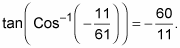##### Trigonometry Workbook For DummiesWhen working with inverse trig functions, it’s always more convenient when the numbers you’re working with are the results of applying one of the trig functions to a common angle measure. When the angle isn’t a common one, though, you need a calculator or table.

By using inverse trig functions, you can solve some interesting problems, where you never even need to know the angle measure. You just need to know a function value, a quadrant, and a few trig identities. For example, you can find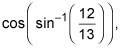which says to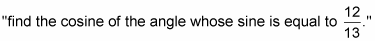You don’t need to know the angle measure to solve this problem, but you do need to know the quadrant that the terminal side lies in, because otherwise, two different angles can be correct answers. The sine is positive in Quadrants I and II, so this problem could involve an angle in either of those quadrants, but cosine isn’t positive in both of those quadrants. Consider the following example.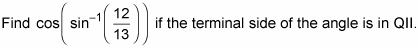1. Use the Pythagorean identity to find the cosine of the angle.

Put the value in for sin θ, get the cosine term alone, and then take the square root of both sides.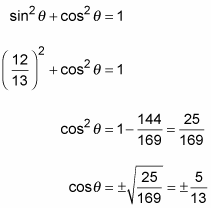2. Choose the sign of the answer.

Because the angle’s terminal side is in QII, and the cosine is negative there, the answer is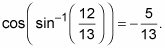The quadrant isn’t a mystery in a problem that uses the inverse trig function. To find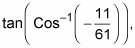you can assume that the angle has its terminal side in QII, because the inverse cosine function is negative in that quadrant.

1. Use the reciprocal identity and reciprocal of the number to find the secant.

The problem involves the angle whose cosine is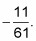Call the unknown angle θ and rewrite the expression in terms of the cosine of θ with that measure. Write the expression this way in order to change from an inverse trig function to a trig function so you can use the identity.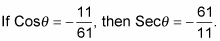2. Use the Pythagorean identity to solve for the tangent.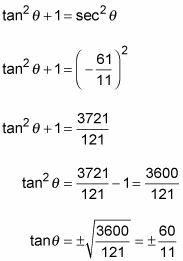3. Choose the sign of the answer.

Because the terminal side is in QII and the tangent is negative in that quadrant,